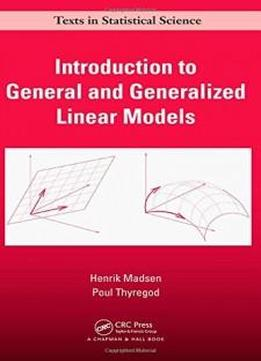# Introduction To General And Generalized Linear Models (chapman & Hall/crc Texts In Statistical Science) by Henrik Madsen / 2010 / English / PDF

Bridging the gap between theory and practice for modern statistical model building,

Bridging the gap between theory and practice for modern statistical model building,Introduction to General and Generalized Linear Models

Introduction to General and Generalized Linear Models presents likelihood-based techniques for statistical modelling using various types of data. Implementations using R are provided throughout the text, although other software packages are also discussed. Numerous examples show how the problems are solved with R.

presents likelihood-based techniques for statistical modelling using various types of data. Implementations using R are provided throughout the text, although other software packages are also discussed. Numerous examples show how the problems are solved with R. After describing the necessary likelihood theory, the book covers both general and generalized linear models using the same likelihood-based methods. It presents the corresponding/parallel results for the general linear models first, since they are easier to understand and often more well known. The authors then explore random effects and mixed effects in a Gaussian context. They also introduce non-Gaussian hierarchical models that are members of the exponential family of distributions. Each chapter contains examples and guidelines for solving the problems via R.

After describing the necessary likelihood theory, the book covers both general and generalized linear models using the same likelihood-based methods. It presents the corresponding/parallel results for the general linear models first, since they are easier to understand and often more well known. The authors then explore random effects and mixed effects in a Gaussian context. They also introduce non-Gaussian hierarchical models that are members of the exponential family of distributions. Each chapter contains examples and guidelines for solving the problems via R. Providing a flexible framework for data analysis and model building, this text focuses on the statistical methods and models that can help predict the expected value of an outcome, dependent, or response variable. It offers a sound introduction to general and generalized linear models using the popular and powerful likelihood techniques. Ancillary materials are available at www.imm.dtu.dk/~hm/GLM

Providing a flexible framework for data analysis and model building, this text focuses on the statistical methods and models that can help predict the expected value of an outcome, dependent, or response variable. It offers a sound introduction to general and generalized linear models using the popular and powerful likelihood techniques. Ancillary materials are available at www.imm.dtu.dk/~hm/GLM

views: 337